Triangle classificationPage 1

WATCH ALL SLIDES

Slide 1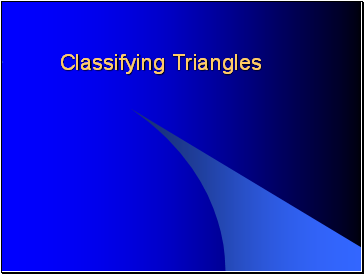Classifying Triangles

Slide 2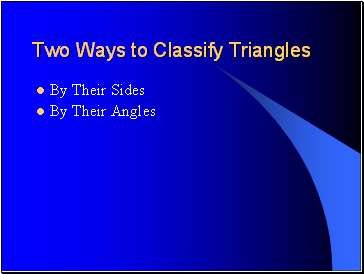Two Ways to Classify Triangles

By Their Sides

By Their Angles

Slide 3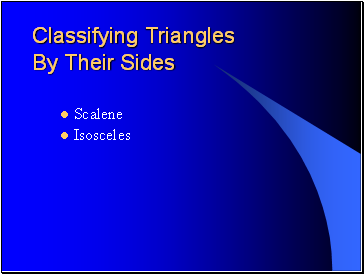Classifying Triangles By Their Sides

Scalene

Isosceles

Slide 4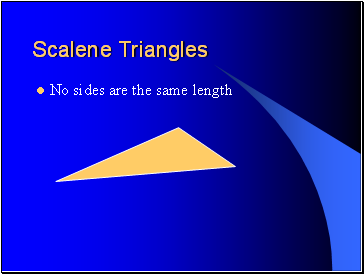Scalene Triangles

No sides are the same length

Slide 5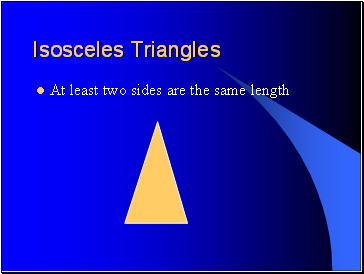Isosceles Triangles

At least two sides are the same length

Slide 6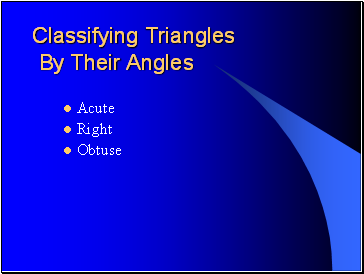Classifying Triangles By Their Angles

Acute

Right

Obtuse

Slide 7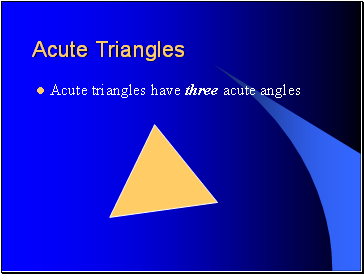Acute Triangles

Acute triangles have three acute angles

Slide 8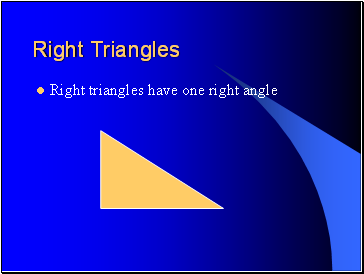Right Triangles

Right triangles have one right angle

Slide 9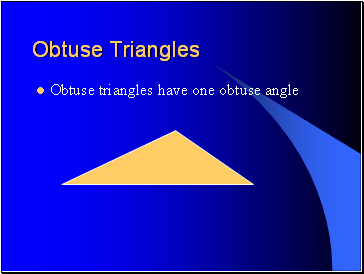Obtuse Triangles

Obtuse triangles have one obtuse angle

Slide 10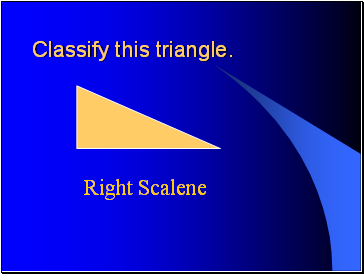Classify this triangle.

Right Scalene

Slide 11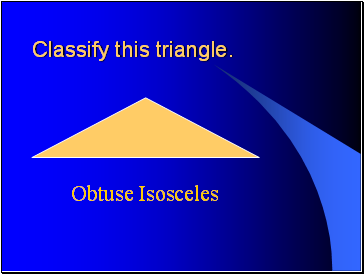Classify this triangle.

Obtuse Isosceles

Slide 12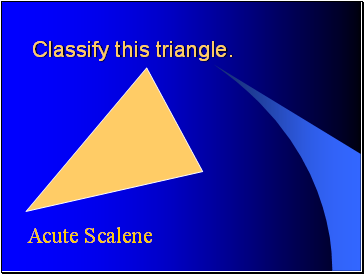Classify this triangle.

Acute Scalene

Slide 13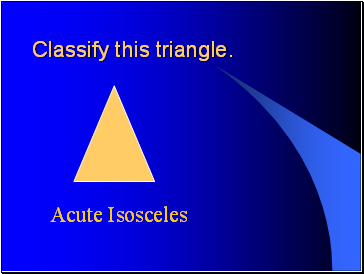Classify this triangle.

Acute Isosceles

Slide 14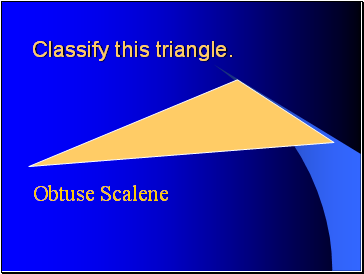Classify this triangle.

Obtuse Scalene

Slide 15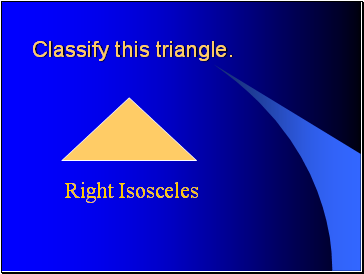Classify this triangle.

Right Isosceles